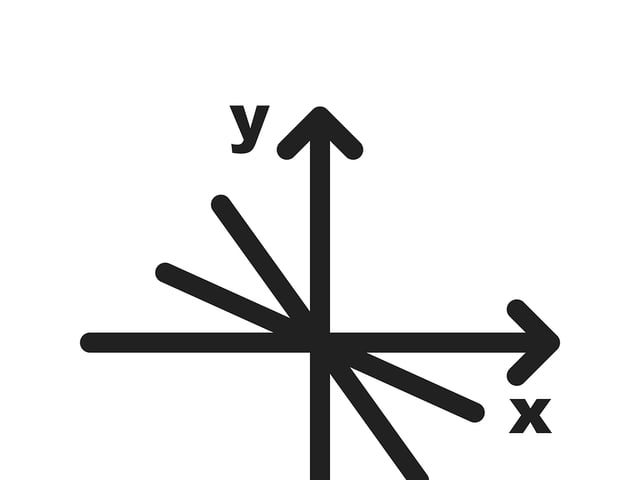# Formulas for the Accuplacer Next Generation Quantitative Reasoning, Algebra, and Statistics Test

The Quantitative Reasoning, Algebra, and Statistics section of the Next Generation Accuplacer test covers a wide range of subjects. It may seem overwhelming when you’re preparing for that test, but we’ve got you covered! In the following chart, you’ll find the essential formulas you’ll need if you want to ace this section. Even though you won’t be able to use them during the test, they will serve as a summary for you. Remember to practice using these formulas to solve the sample problems we have prepared for you, and also to read our study guide for more developed content here at Union Test Prep!

## Exponents

Formula Symbols
$$x^a \cdot x^b = x^{a+b}$$ $$a, b, x =\text{any real number}$$
$$\dfrac{x^a}{x^b}=x^{a-b}$$ $$a, b, x =\text{any real number}$$
$$(x^a)^b=x^{a \cdot b}$$ $$a, b, x =\text{any real number}$$
$$(x \cdot y)^a=x^a \cdot y^a$$ $$a, x, y =\text{any real number}$$
$$x^1=x$$ $$x= \text{any real number}$$
$$x^0 = 1$$ $$x = \text{any real number}$$
$$x^{-a} = \dfrac{1}{x^a}$$ $$a,x = \text{any real number}$$
$$x^{\frac{a}{b}} = \sqrt[b]{x^a} = (\sqrt[b]{x})^a$$ $$a, b, x = \text{any real number}$$

## Logarithms

Formula Symbols
$$a^x = b \Rightarrow \log_a{b}=x$$ $$a, b, x = \text{any real number}$$
$$\ln(x) = \log_e x$$ $$x = \text{any real number}$$
$$e = \text{Euler's number} \approx 2.718$$
$$a^{\log_a x}=x$$ $$a, x = \text{any real number}$$
$$\log(a \cdot b) = \log(a) + \log(b)$$ $$a, b = \text{any real number}$$
$$\log(a \div b) = \log(a) - \log(b)$$ $$a, b = \text{any real number}$$
$$\log(a^b) = b \cdot \log(a)$$ $$a, b = \text{any real number}$$
$$\log_a x = \log_b x \cdot \log_a b$$ $$a, b, x = \text{any real number}$$
$$\log_a b = \dfrac{\log_x b}{\log_x a}$$ $$a, b,x = \text{any real number}$$
$$\log_a a = 1$$ $$a = \text{any real number}$$
$$\log(1) = 0$$

## Linear Equations

$$A\cdot x + B\cdot y = C$$ $$A, B, C = \text{any real number}$$
$$y= \text{dependent variable}$$
$$x = \text{independent variable}$$
Standard Form
$$y=m \cdot x + b$$ $$y = \text{dependent variable}$$
$$m= \text{slope}$$
$$x = \text{independent variable}$$
$$b = y \text{-intercept}$$
Slope-Intercept Form. Try to convert linear equations to this format.
$$m = \dfrac{y_2 - y_1}{x_2 - x_1}$$ $$m = \text{slope}$$
$$y_n = \text{dependent variable (point n)}$$
$$x_n = \text{independent variable (point n)}$$
This is a rearranged version of the point-slope form.
$$y-y_1 = m(x-x_1)$$ $$y= \text{dependent variable}$$
$$x = \text{independent variable}$$
$$y_1 = y \text{ value of a point on the line}$$
$$x_1 = x \text{ value of a point on the line}$$
$$m = \text{slope}$$
Point-Slope form
$$x+a = b \Rightarrow x = b-a$$
$$x-a = b \Rightarrow x = b+a$$
$$x \cdot a = b \Rightarrow x = b \div a$$
$$x \div a = b \Rightarrow x = b \cdot a$$
$$x^a = b \Rightarrow x = \sqrt[a]{b}$$
$$\sqrt[a]{x} = b \Rightarrow x = b^a$$
$$a^x = b \Rightarrow x = \dfrac{\log b}{\log a}$$
$$a, b = \text{constants}$$
$$x = \text{variable}$$

## Statistics

Formula Symbols
$$p = \dfrac{d}{t}$$ $$p = \text{probability of an event}$$
$$d = \text{desired event}$$
$$t= \text{total number of possible events}$$
$$\bar{x} = \dfrac{\sum{x_i}}{n}$$ $$\bar{x} = \text{mean}$$
$$x_i = \text{value of each measurement}$$
$$n = \text{number of measurements}$$
$$s = \sqrt{\mathstrut \dfrac{\sum(x_i - \bar{x})^2}{n-1}}$$ $$s = \text{standard deviation}$$
$$\bar{x}=\text{mean}$$
$$x_i = \text{value of each measurement}$$
$$n = \text{number of measurements}$$
$$V = s^2$$ $$V = \text{variance}$$
$$s = \text{standard deviation}$$
$$CV = RSD = 100 \cdot \dfrac{s}{\bar{x}}$$ $$CV = \text{coefficient of variation}$$
$$RSD = \text{relative standard deviation}$$
$$s = \text{standard deviation}$$
$$\bar{x} = \text{mean}$$

## Geometry

$$A = s^2$$ $$A = \text{area of a square}$$
$$s = \text{side length}$$

$$A = l \cdot w$$ $$A = \text{area of a rectangle}$$
$$l = \text{length}$$
$$w = \text{width}$$

$$A = \dfrac{1}{2} b \cdot h$$ $$A = \text{area of a triangle}$$
$$b= \text{base}$$
$$h = \text{height}$$

$$A = \pi \cdot r^2$$ $$A = \text{area of a circle}$$
$$r = \text{radius}$$

$$A = h \cdot \dfrac{b_1+b_2}{2}$$ $$A = \text{area of a trapezoid}$$
$$b_n = \text{base }n$$
$$h = \text{height}$$

$$C= 2 \pi r = \pi d$$ $$C = \text{perimeter of a circle}$$
$$r = \text{radius}$$
$$d = \text{diameter}$$

$$V = s^3$$ $$V = \text{volume of a cube}$$
$$s = \text{side length}$$

$$V = l \cdot w \cdot h$$ $$V = \text{volume of a rectangular prism}$$
$$l = \text{length}$$
$$w = \text{width}$$
$$h = \text{height}$$

$$V = \dfrac{4}{3} \pi r^3$$ $$V = \text{volume of a sphere}$$
$$r = \text{radius}$$

$$V = \pi r^2 h$$ $$V = \text{volume of a cylinder}$$
$$r = \text{radius of base}$$
$$h = \text{height}$$

$$V = \dfrac{1}{3} \pi r^2 h$$ $$V = \text{volume of a cone}$$
$$r = \text{radius}$$
$$h = \text{height}$$

$$V = \dfrac{1}{3} l \cdot w \cdot h$$ $$V = \text{volume of a pyramid}$$
$$l = \text{length}$$
$$w = \text{width}$$
$$h = \text{height}$$

$$d= \sqrt{\mathstrut (y_2 - y_1)^2 + (x_2-x_1)^2}$$ $$d = \text{distance between two points}$$
$$y_n = y \text{ value at point n}$$
$$x_n = x \text{ value at point n}$$

$$a^2 + b^ 2 = c^ 2$$ $$a,b = \text{legs of a right triangle}$$
$$c = \text{hypotenuse of a right triangle}$$
Pythagorean theorem
$$(x-h)^2 + (y-k)^2 = r^2$$ $$(h,k) = \text{center of a circle}$$
$$r = \text{radius}$$
Standard form of a circle
$$x^2 + y^2 + Ax + By + C = 0$$ $$x, y = \text{variables}$$
$$A,B,C = \text{constants}$$
General form of a circle